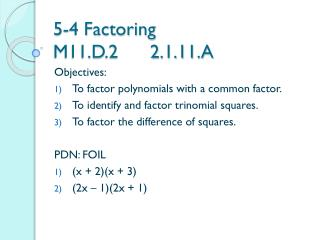DownloadDownload Presentation5-4 Factoring M11.D.2 2.1.11.A

# 5-4 Factoring M11.D.2 2.1.11.A

Télécharger la présentation## 5-4 Factoring M11.D.2 2.1.11.A

- - - - - - - - - - - - - - - - - - - - - - - - - - - E N D - - - - - - - - - - - - - - - - - - - - - - - - - - -
##### Presentation Transcript

1. 5-4 FactoringM11.D.2 2.1.11.A Objectives: To factor polynomials with a common factor. To identify and factor trinomial squares. To factor the difference of squares. PDN: FOIL (x + 2)(x + 3) (2x – 1)(2x + 1)

2. Vocab • To factor an expression means to write it as an equivalent expression that is a product. Factoring is the reverse of multiplying.

3. Examples: Factor out a common factor • 3x + 12 • 3x² + 12 • 7y³ + 14y² • 6u²v³ + 21uv²

4. Try This Pg. 219 a-c

5. Vocab • Greatest Common Factor (gcf) – factor out all common factors, both numbers and variables.

6. Examples: Factor when a = 1 • x² + 6x + 8 • x² -7x + 10 • x² + 4x - 5

7. Factor when a = 1 • x² - 4x -5 • x² -8x + 16 • x² + 8x + 16

8. Try This Pg. 220 d

9. Examples: Factor • x² - 25 • 9x² - 16y² • 64x - 16y²

10. Try This Pg. 221 j - l

11. Examples: Factor by Grouping • x² + 3x + 2x + 6 • ac + ad + bc + bd • ax – 3a + bx – 3b